## RS Aggarwal Class 8 Solutions Chapter 18 Area of a Trapezium and a Polygon Ex 18B

These Solutions are part of RS Aggarwal Solutions Class 8. Here we have given RS Aggarwal Solutions Class 8 Chapter 18 Area of a Trapezium and a Polygon Ex 18B.

Other Exercises

Question 1.
Solution:
AC = 24 cm, BL ⊥ AC and DM ⊥ AC
BL = 8 cm and DM = 7 cm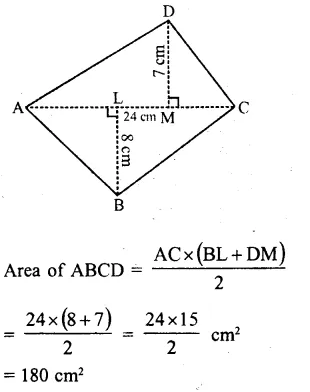Question 2.
Solution:
In quad. ABCD, diagonal BD = 36 m
AL ⊥ BD and CM ⊥ BD
AL = 19 m and CM = 11 mQuestion 3.
Solution:
In the given pentagon ABCDE,
BL ⊥ AC, DM ⊥ AC, EN ⊥ AC
AC = 18 cm, AM = 14 cm, AN = 6 cm,
BL = 4 cm, DM = 12 cm and EN = 9 cm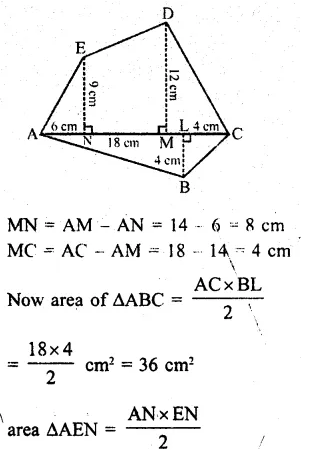Question 4.
Solution:
In hexagon ABCDEF, there are triangles and trapeziums
AP = 6 cm, PL = 2 cm, LN = 8 cm,
NM = 2 cm, MD = 3 cm, FP = 8 cm,
EN = 12 cm, BL = 8 cm and CM = 6 cm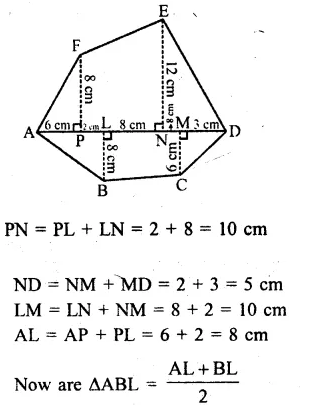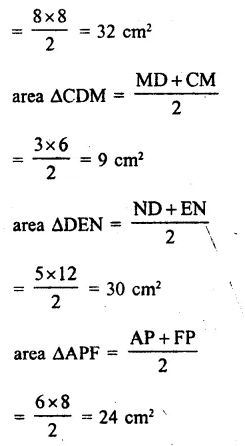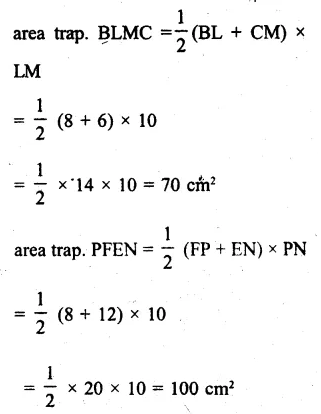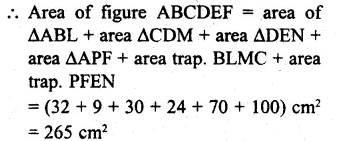Question 5.
Solution:
In the given pentagon ABCDE,
AC = 10 cm, D = 12 cm, BL = 3 cm,
CM = 7 cm and EN = 5 cm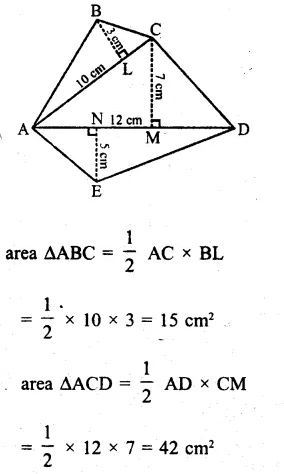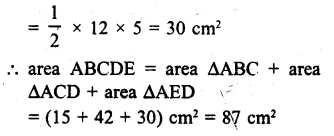Question 6.
Solution:
In the figure, ABCF is 0 square and CDEF is a trapezium
Now area of sq. ABCF
= (side)² = (20)² = 400 cm²
area of trap. CDEF
= $$\\ \frac { 1 }{ 2 }$$ (ED + FC ) x height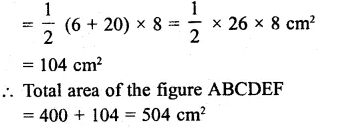Question 7.
Solution:
In the right ∆ABC
AB² = BC² + AC²
=> (5)² = (4)² + AC²
25 = 16 + AC²
AC² = 25 – 16 = 9 = (3)²
AC = 3 cm= 32 + 36
= 68 cm²

Question 8.
Solution:
AD = 23 cm, LM = 13 cm
AL = MD = $$\\ \frac { 23-13 }{ 2 }$$ = $$\\ \frac { 10 }{ 2 }$$ = 5 cm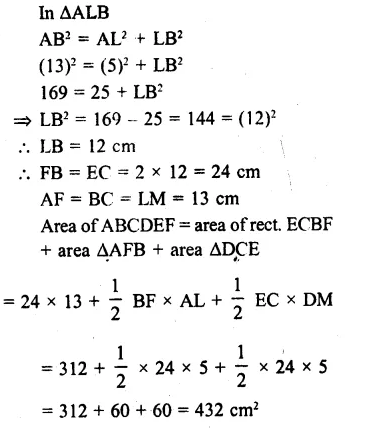Hope given RS Aggarwal Solutions Class 8 Chapter 18 Area of a Trapezium and a Polygon Ex 18B are helpful to complete your math homework.

If you have any doubts, please comment below. Learn Insta try to provide online math tutoring for you.